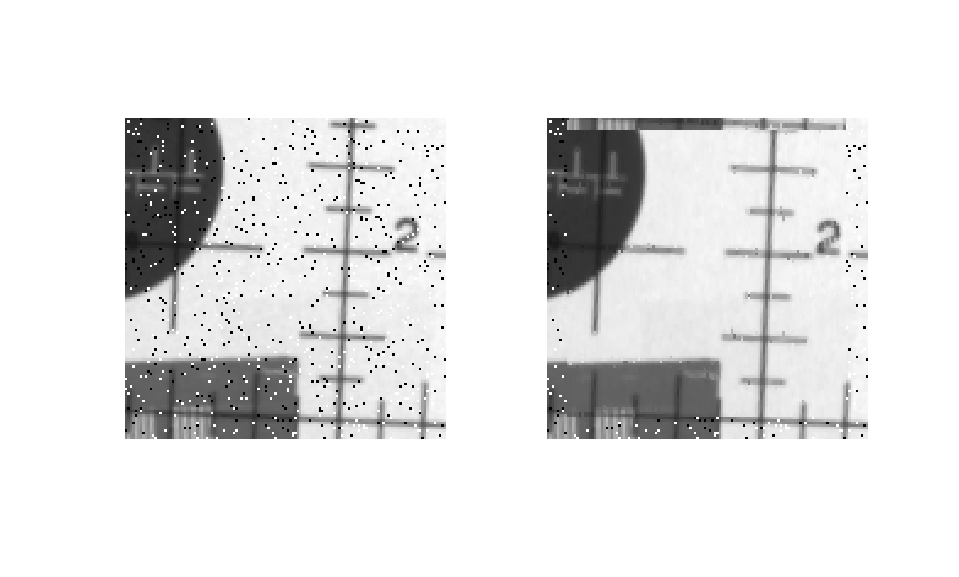# HDL Code Generation for Adaptive Median Filter

This example shows how to generate HDL code from a MATLAB® design that implements an adaptive median filter algorithm and generates HDL code.

An adaptive median filter performs spatial processing to reduce noise in an image. The filter compares each pixel in the image to the surrounding pixels. If one of the pixel values differ significantly from the majority of the surrounding pixels, the pixel is treated as noise. The filtering algorithm then replaces the noise pixel by the median values of the surrounding pixels. This process repeats until all noise pixels in the image are removed.

```design_name = 'mlhdlc_median_filter'; testbench_name = 'mlhdlc_median_filter_tb'; ```

Review the MATLAB design:

```edit(design_name); ```
```%#codegen function [pixel_val, pixel_valid] = mlhdlc_median_filter(c_data, c_idx) % Copyright 2011-2019 The MathWorks, Inc. smax = 9; persistent window; if isempty(window) window = zeros(smax, smax); end cp = ceil(smax/2); % center pixel; w3 = -1:1; w5 = -2:2; w7 = -3:3; w9 = -4:4; r3 = cp + w3; % 3x3 window r5 = cp + w5; % 5x5 window r7 = cp + w7; % 7x7 window r9 = cp + w9; % 9x9 window d3x3 = window(r3, r3); d5x5 = window(r5, r5); d7x7 = window(r7, r7); d9x9 = window(r9, r9); center_pixel = window(cp, cp); % use 1D filter for 3x3 region outbuf = get_median_1d(d3x3(:)'); [min3, med3, max3] = getMinMaxMed_1d(outbuf); % use 2D filter for 5x5 region outbuf = get_median_2d(d5x5); [min5, med5, max5] = getMinMaxMed_2d(outbuf); % use 2D filter for 7x7 region outbuf = get_median_2d(d7x7); [min7, med7, max7] = getMinMaxMed_2d(outbuf); % use 2D filter for 9x9 region outbuf = get_median_2d(d9x9); [min9, med9, max9] = getMinMaxMed_2d(outbuf); pixel_val = get_new_pixel(min3, med3, max3, ... min5, med5, max5, ... min7, med7, max7, ... min9, med9, max9, ... center_pixel); % we need to wait until 9 cycles for the buffer to fill up % output is not valid every time we start from col1 for 9 cycles. persistent datavalid if isempty(datavalid) datavalid = false; end pixel_valid = datavalid; datavalid = (c_idx >= smax); % build the 9x9 buffer window(:,2:smax) = window(:,1:smax-1); window(:,1) = c_data; end %%%%%%%%%%%%%%%%%%%%%%%%%%%%%%%%%%%%%%%%%%%%%%%%%%%%%%%%%%%%%%%%%%%%%%%%%%% function [min, med, max] = getMinMaxMed_1d(inbuf) max = inbuf(1); med = inbuf(ceil(numel(inbuf)/2)); min = inbuf(numel(inbuf)); end %%%%%%%%%%%%%%%%%%%%%%%%%%%%%%%%%%%%%%%%%%%%%%%%%%%%%%%%%%%%%%%%%%%%%%%%%%% function [min, med, max] = getMinMaxMed_2d(inbuf) [nrows, ncols] = size(inbuf); max = inbuf(1, 1); med = inbuf(ceil(nrows/2), ceil(ncols/2)); min = inbuf(nrows, ncols); end %%%%%%%%%%%%%%%%%%%%%%%%%%%%%%%%%%%%%%%%%%%%%%%%%%%%%%%%%%%%%%%%%%%%%%%%%%% function new_pixel = get_new_pixel(... min3, med3, max3, ... min5, med5, max5, ... min7, med7, max7, ... min9, med9, max9, ... center_data) if (med3 > min3 && med3 < max3) new_pixel = get_center_data(min3, med3, max3,center_data); elseif (med5 > min5 && med5 < max5) new_pixel = get_center_data(min5, med5, max5,center_data); elseif (med7 > min7 && med7 < max7) new_pixel = get_center_data(min7, med7, max7,center_data); elseif (med9 > min9 && med9 < max9) new_pixel = get_center_data(min9, med9, max9,center_data); else new_pixel = center_data; end end %%%%%%%%%%%%%%%%%%%%%%%%%%%%%%%%%%%%%%%%%%%%%%%%%%%%%%%%%%%%%%%%%%%%%%%%%%% function [new_data] = get_center_data(min,med,max,center_data) if center_data <= min || center_data >= max new_data = med; else new_data = center_data; end end %%%%%%%%%%%%%%%%%%%%%%%%%%%%%%%%%%%%%%%%%%%%%%%%%%%%%%%%%%%%%%%%%%%%%%%%%%% % perform median 1d computation %%%%%%%%%%%%%%%%%%%%%%%%%%%%%%%%%%%%%%%%%%%%%%%%%%%%%%%%%%%%%%%%%%%%%%%%%%% function outbuf = get_median_1d(inbuf) numpixels = length(inbuf); tbuf = inbuf; for ii=coder.unroll(1:numpixels) if bitand(ii,uint32(1)) == 1 tbuf = compare_stage1(tbuf); else tbuf = compare_stage2(tbuf); end end outbuf = tbuf; end function outbuf = compare_stage1(inbuf) numpixels = length(inbuf); tbuf = compare_stage(inbuf(1:numpixels-1)); outbuf = [tbuf(:)' inbuf(numpixels)]; end function outbuf = compare_stage2(inbuf) numpixels = length(inbuf); tbuf = compare_stage(inbuf(2:numpixels)); outbuf = [inbuf(1) tbuf(:)']; end function [outbuf] = compare_stage(inbuf) step = 2; numpixels = length(inbuf); outbuf = inbuf; for ii=coder.unroll(1:step:numpixels) t = compare_pixels([inbuf(ii), inbuf(ii+1)]); outbuf(ii) = t(1); outbuf(ii+1) = t(2); end end function outbuf = compare_pixels(inbuf) if (inbuf(1) > inbuf(2)) outbuf = [inbuf(1), inbuf(2)]; else outbuf = [inbuf(2), inbuf(1)]; end end %%%%%%%%%%%%%%%%%%%%%%%%%%%%%%%%%%%%%%%%%%%%%%%%%%%%%%%%%%%%%%%%%%%%%%%%%%% % perform median 2d computation %%%%%%%%%%%%%%%%%%%%%%%%%%%%%%%%%%%%%%%%%%%%%%%%%%%%%%%%%%%%%%%%%%%%%%%%%%% function outbuf = get_median_2d(inbuf) outbuf = inbuf; [nrows, ncols] = size(inbuf); for ii=coder.unroll(1:ncols) colData = outbuf(:, ii)'; colDataOut = get_median_1d(colData)'; outbuf(:, ii) = colDataOut; end for ii=coder.unroll(1:nrows) rowData = outbuf(ii, :); rowDataOut = get_median_1d(rowData); outbuf(ii, :) = rowDataOut; end end ```

The MATLAB function is modular and uses several functions to filter the noise in the image.

### Adaptive Filter MATLAB Test Bench

A MATLAB test bench `mlhdlc_median_filter_tb` exercises the filter design by using a representative input range.

Review the MATLAB test bench:

```edit(testbench_name); ```
```I = imread('mlhdlc_img_pattern_noisy.tif'); J = I; % Copyright 2011-2019 The MathWorks, Inc. smax = 9; [nrows, ncols] = size(I); ll = ceil(smax/2); ul = floor(smax/2); for ii=1:ncols-smax for jj=1:nrows-smax c_idx = ii; c_data = double(I(jj:jj+smax-1, ii)); [pixel_val, pixel_valid] = mlhdlc_median_filter(c_data, c_idx); if pixel_valid J(jj, ii) = pixel_val; end end end h = figure; set( h, 'Name', [ mfilename, '_plot' ] ); subplot( 1, 2, 1 ); imshow( I, [ ] ); subplot( 1, 2, 2 ); imshow( J, [ ] ); ```

### Test the MATLAB Algorithm

To avoid run-time errors, simulate the design with the test bench.

```mlhdlc_median_filter_tb ```### Accelerating the Design for Faster Simulation

To simulate the test bench faster:

1. Create a MEX file by using MATLAB Coder™. The HDL Workflow Advisor automates these steps when running fixed-point simulations of the design.

``` codegen -o mlhdlc_median_filter -args {zeros(9,1), 0} mlhdlc_median_filter [~, tbn] = fileparts(testbench_name);```

2. Simulate the design by using the MEX file. When you run the test bench, HDL Coder uses the MEX file and runs the simulation faster.

` mlhdlc_median_filter_tb`

3. Clean up the MEX file.

``` clear mex; rmdir('codegen', 's'); delete(['mlhdlc_median_filter', '.', mexext]);```

### Create an HDL Coder Project

1. Create an HDL Coder project:

```coder -hdlcoder -new mlhdlc_med_filt_prj ```

2. Add the file `mlhdlc_median_filter.m` to the project as the MATLAB Function and `mlhdlc_median_filter_tb.m` as the MATLAB Test Bench.

3. Click Autodefine types and use the recommended types for the inputs and outputs of the MATLAB function `mlhdlc_median_filter`.Refer to Get Started with MATLAB to HDL Workflow for a more complete tutorial on creating and populating MATLAB HDL Coder projects.

### Run Fixed-Point Conversion and HDL Code Generation

A single HDL file `mlhdlc_median_filter_fixpt.vhd` is generated for the MATLAB design. To examine the generated HDL code for the filter design, click the hyperlinks in the Code Generation Log window.